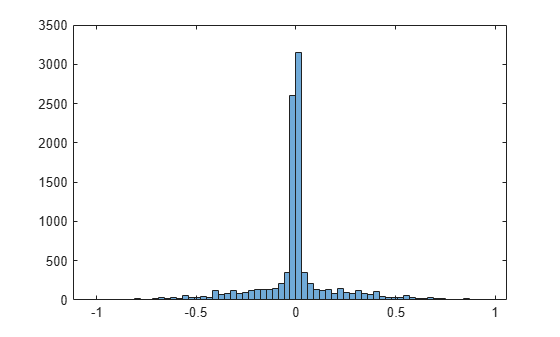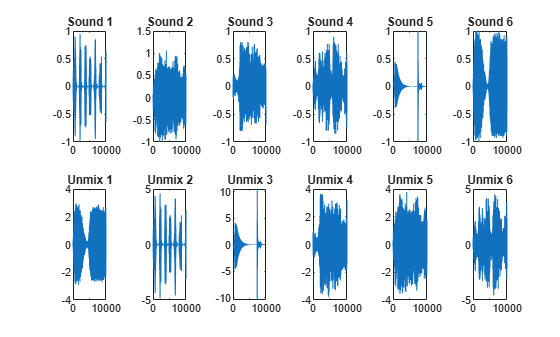# Extract Mixed Signals

This example shows how to use `rica` to disentangle mixed audio signals. You can use `rica` to perform independent component analysis (ICA) when prewhitening is included as a preprocessing step. The ICA model is

`$x=\mu +As.$`

Here, $x$ is a $p$-by-1 vector of mixed signals, $\mu$ is a $p$-by-1 vector of offset values, $A$ is a $p$-by- $q$ mixing matrix, and $s$ is a $q$-by-1 vector of original signals. Suppose first that $A$ is a square matrix. If you know $\mu$ and $A$, you can recover an original signal $s$ from the data $x$:

`$s={A}^{-1}\left(x-\mu \right).$`

Using the `rica` function, you can perform this recovery even without knowing the mixing matrix $A$ or the mean $\mu$. Given a set of several observations $x\left(1\right)$, $x\left(2\right)$, ..., `rica` extracts the original signals $s\left(1\right)$, $s\left(2\right)$, ....

Load a set of six audio files, which ship with MATLAB®. Trim each file to 10,000 samples.

```files = {'chirp.mat' 'gong.mat' 'handel.mat' 'laughter.mat' 'splat.mat' 'train.mat'}; S = zeros(10000,6); for i = 1:6 test = load(files{i}); y = test.y(1:10000,1); S(:,i) = y; end```

### Mix Signals

Mix the signals together by using a random mixing matrix and add a random offset.

```rng('default') % For reproducibility mixdata = S*randn(6) + randn(1,6);```

To listen to the original sounds, execute this code:

``` for i = 1:6 disp(i); sound(S(:,i)); pause; end ```

To listen to the mixed sounds, execute this code:

``` for i = 1:6 disp(i); sound(mixdata(:,i)); pause; end ```

Plot the signals.

```figure tiledlayout(2,6) for i = 1:6 nexttile(i) plot(S(:,i)) title(['Sound ',num2str(i)]) nexttile(i+6) plot(mixdata(:,i)) title(['Mix ',num2str(i)]) end```The original signals have clear structure. The mixed signals have much less structure.

### Prewhiten Mixed Signals

To separate the signals effectively, "prewhiten" the signals by using the `prewhiten` function that appears at the end of this example. This function transforms `mixdata` so that it has zero mean and identity covariance.

The idea is the following. If $s$ is a zero-mean source with statistically independent components, then

`$E\left(s\right)=0$`

`$E\left(s{s}^{T}\right)=I.$`

Then the mean and covariance of $x$ are

`$E\left(x\right)=\mu$`

`$Cov\left(x\right)=A{A}^{T}=C.$`

Suppose that you know $\mu$ and $C$. In practice, you would estimate these quantities from the sample mean and covariance of the columns of $x$. You can solve for $s$ in terms of $x$ by

`$s={A}^{-1}\left(x-\mu \right)=\left({A}^{T}A{\right)}^{-1}{A}^{T}\left(x-\mu \right).$`

The latter equation holds even when $A$ is not a square invertible matrix.

Suppose that $U$ is a $p$-by- $q$ matrix of left eigenvectors of the positive semidefinite matrix $C$, and $\Sigma$ is the $q$-by- $q$ matrix of eigenvalues. Then

`$C=U\Sigma {U}^{T}$`

`${U}^{T}U=I.$`

Then

`$A{A}^{T}=U\Sigma {U}^{T}.$`

There are many mixing matrices $A$ that satisfy this last equation. If $W$ is a $q$-by- $q$ orthonormal matrix, then

`${W}^{T}W=W{W}^{T}=I$`

`$A=U{\Sigma }^{1/2}W.$`

Substituting into the equation for $s$,

`$s={W}^{T}\underset{}{\overset{\sim }{x}},$`

where

`$\underset{}{\overset{\sim }{x}}={\Sigma }^{-1/2}{U}^{T}\left(x-\mu \right).$`

$\underset{}{\overset{\sim }{x}}$ is the prewhitened data. `rica` computes the unknown matrix $W$ under the assumption that the components of $s$ are as independent as possible.

`mixdata = prewhiten(mixdata);`

### Separate All Signals

A super-Gaussian source has a sharp peak near zero, such as a histogram of sound 1 shows.

```figure histogram(S(:,1))```Perform Reconstruction ICA while asking for six features. Indicate that each source is super-Gaussian.

```q = 6; Mdl = rica(mixdata,q,'NonGaussianityIndicator',ones(6,1));```

Extract the features. If the unmixing procedure is successful, the features are proportional to the original signals.

`unmixed = transform(Mdl,mixdata);`

### Compare Unmixed Signals To Original Signals

Plot the original and unmixed signals.

```figure tiledlayout(2,6) for i = 1:6 nexttile(i) plot(S(:,i)) title(['Sound ',num2str(i)]) nexttile(i+6) plot(unmixed(:,i)) title(['Unmix ',num2str(i)]) end```The order of the unmixed signals is different than the original order. Reorder the columns so that the unmixed signals match the corresponding original signals. Scale the unmixed signals to have the same norms as the corresponding original signals. (`rica` cannot identify the scale of the original signals because any scale can lead to the same signal mixture.)

```unmixed = unmixed(:,[2,5,4,6,3,1]); for i = 1:6 unmixed(:,i) = unmixed(:,i)/norm(unmixed(:,i))*norm(S(:,i)); end```

Plot the original and unmixed signals.

```figure tiledlayout(2,6) for i = 1:6 nexttile(i) plot(S(:,i)) ylim([-1,1]) title(['Sound ',num2str(i)]) nexttile(i+6) plot(unmixed(:,i)) ylim([-1,1]) title(['Unmix ',num2str(i)]) end```The unmixed signals look similar to the original signals. To listen to the unmixed sounds, execute this code.

``` for i = 1:6 disp(i); sound(unmixed(:,i)); pause; end ```

Here is the code for the `prewhiten` function.

```function Z = prewhiten(X) % X = N-by-P matrix for N observations and P predictors % Z = N-by-P prewhitened matrix % 1. Size of X. [N,P] = size(X); assert(N >= P); % 2. SVD of covariance of X. We could also use svd(X) to proceed but N % can be large and so we sacrifice some accuracy for speed. [U,Sig] = svd(cov(X)); Sig = diag(Sig); Sig = Sig(:)'; % 3. Figure out which values of Sig are non-zero. tol = eps(class(X)); idx = (Sig > max(Sig)*tol); assert(~all(idx == 0)); % 4. Get the non-zero elements of Sig and corresponding columns of U. Sig = Sig(idx); U = U(:,idx); % 5. Compute prewhitened data. mu = mean(X,1); Z = X-mu; Z = (Z*U)./sqrt(Sig); end```